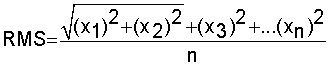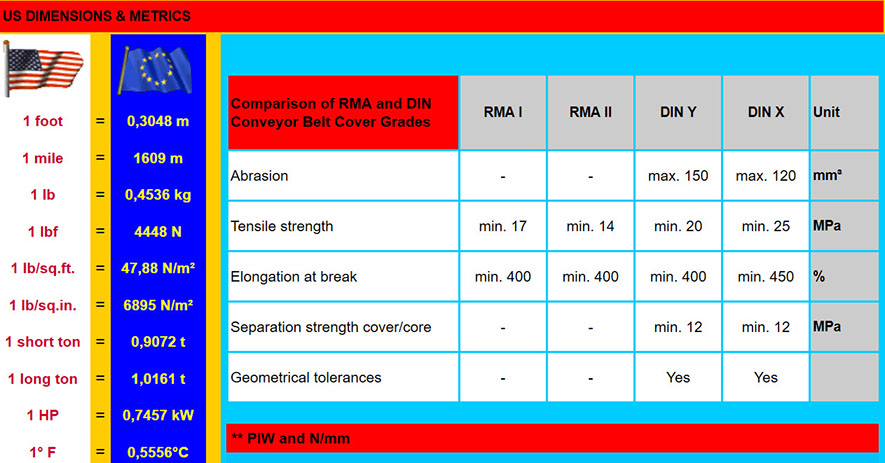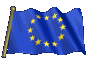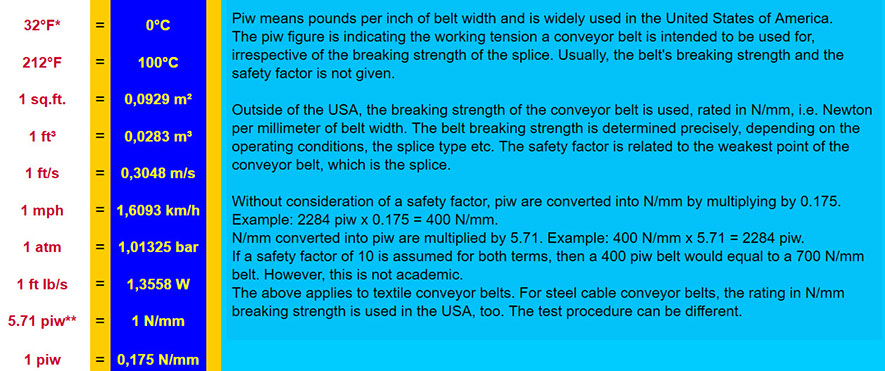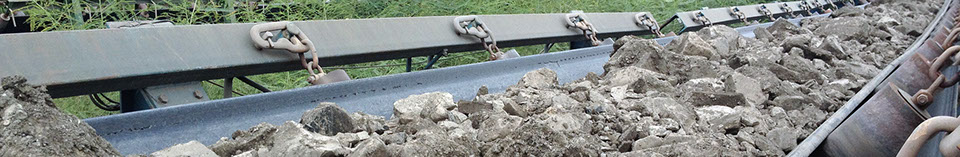EQUATIONS

The troughability of a conveyor belt can be estimated by using this equation,

where

m"G = belt mass in kg/m²

B = belt width in m

Sz = carcass thickness in mm

Cq = transverse rigidity factor (polyamide = 18, steel cord = 42)

Test

TROUGHABILITY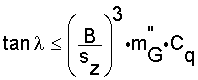The modulus of elasticity is calculated by dividing the stress by the strain,

where

M = modulus of elasticity (ISO 9856)

F = force (N)

εelast = elastic elongation at the end of the specified number of cycles (N/mm)

In other words: The higher the modulus the lower the elastic elongation per unit stress. More

MODULUS OF ELASTICITY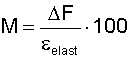The modulus of elasticity can be used to calculate the tension force it exerts under a specific extension,

where

T = tension force

λ = modulus of elasticity

A = cross-sectional area

x = extension

l = length (m)

TENSION FORCE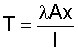The minimum belt tensions for transmitting

the pulley peripheral forces are calculated as follows,

where

Fu = minimum peripheral force

C = coefficient C

f = artificial friction coefficient

L = conveyor length (m)

g = acceleration (m/s²)

qRo mass of revolving idler parts of top strand (kg/m),

qRu mass of revolving idler parts of bottom strand (kg/m),

qB mass of the belt on top strand (kg/m),

qG mass of the belt in bottom strand (kg/m),

FS1 special main resistances,

FS2 special secondary resistances

Chart

MINIMUM PERIPHERAL FORCE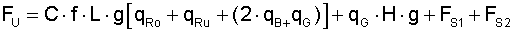The required take-up length is calculated as follows,

where

SSp = take-up length (m)

L = conveyor length (m)

ε = belt elongation, elastic and permanent (%)

As a rough guideline, use 1,5 % elongation for textile belts

and 0,2 % for steel cord belts.

Note: For long-distance conveyors, dynamic start-up calculations

may be required, because not all elements are set in motion simultaneously,

due to the elastic properties of the conveyor belt.

TAKE-UP LENGTH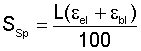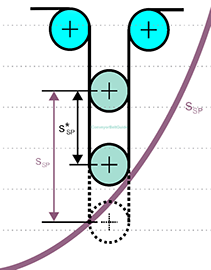The coefficient C is a function of the length of the conveyor.

The total resistances without slope and special resistances are divided by the main resistances,

where

C = coefficient C

FH = primary resistances

FN = secondary resistances

Chart

COEFFICIENT CThe Arrhenius equation describes the quantitative relation between reaction velocity and temperature

(the speed of chemical reactions increases with rising temperature),

where

k = temperature dependence of the rate constant (of a chemical reaction)

EA = activation energy

T = temperature (in Kelvin)

R = gas constant

Ae = prefactor (frequency factor)

ARRHENIUS EQUATIONwhere

σ = stress

v = period of strain oscillation

δ = phase lag between stress and strain

STRESS IN RUBBER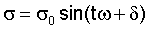where

ε = strain

ω = period of strain oscillation

t = time

STRAIN IN RUBBER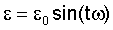The storage modulus measures the stored energy, representing the elastic portion,

and the energy dissipated as heat, representing the viscous portion,

where

E' = storage modulus

σ = stress

ε = strain

δ = phase lag between stress and strain

STORAGE MODULUSThe loss modulus measures the stored energy, representing the elastic portion,

and the energy dissipated as heat, representing the viscous portion,

where

E'' = loss modulus

σ = stress

ε = strain

δ = phase lag between stress and strain

LOSS MODULUS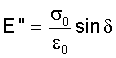Internal friction is the force resisting motion between the elements making up a solid material

while it undergoes deformation. The tan δ is sometimes used to determine the indentation

loss of a conveyor belt cover (energy saving belts). E' and E'' should be as low as possible.

However, there are a number of misconceptions related to specifying E' and E''.

Where

tan δ = internal friction of a rubber

E' = storage modulus (N/mm²)

E'' = loss modulus (N/mm²)

INTERNAL FRICTION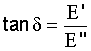Where

v = belt velocity (m/s),

lvth = theoretical volume flow (m³/h),

ρ = bulk density of the conveyed material (t/m³),

φSt = coefficient for determination of the volume flow.

More

LENGTH RELATED MASS FLOW (m³/h)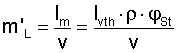Where,

PB0 = braking factor related to the rated torque of all drive motors,

ηges = overall efficiency of all transmission elements between motor and pulley shaft,

PMerf = total capacity of the drive motors required in a steady operating state,

PMinst is the total installed capacity of the drive motors (N).

BRAKING FACTOR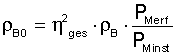Where

g = gravity (9,81 m/s²)

m'Li = mass of the conveyed material, uniformly distributed across a section of the conveyor (kg/m)

m'G = length related mass of the conveyor belt (kg/m)

IRo = idler spacing in top run (m)

hrel = maximum belt sag related to the spacing between the carry idlers (%)

MINIMUM BELT TENSION FOR BELT SAG LIMITATION (top side, loaded)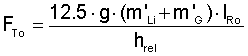Where

g = gravity (9,81 m/s²)

m'G = length related mass of the conveyor belt (kg/m)

IRu = idler spacing in bottom run (m)

hrel = maximum belt sag related to the spacing between the carry idlers (%)

MINIMUM BELT TENSION FOR BELT SAG LIMITATION (bottom side, unloaded)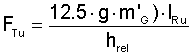Where

f = friction factor in top and bottom run

L = conveyor length (m)

g = gravity acceleration (m/s²)

m'R = mass of the idlers (kg/m)

m'G = length related mass of the conveyor belt in both runs (kg/m)

m'L = mass of the conveyor belt with an evenly distributed load (kg/m)

δ = even inclination of the conveyor (°)

PRIMARY RESISTANCES IN AN EVENLY TILTED CONVEYOR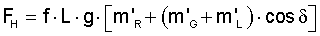The Voigt model consists of a Newtonian damper and Hookean elastic spring connected in parallel.

It is used to explain the creep resp. relaxation behaviour of polymers.

Where

η = dynamic viscosity

τ = total stress

γ = total deformation

D = shear rate

G = shear modulus

VOIGT MODEL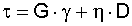Where

C = coefficient (main resistance factor)

f = resistance coefficient

L = belt length (m)

g = acceleration (m/s²)

qRO = mass of the idlers on top side (kg/m)

qRU = mass of the idlers on bottom side (kg/m)

qB = belt mass (kg/m)

qG = mass of the conveyed material (kg/m)

H = lift (m)

FS1 = special main resistances

FS2 = special secondary resistances

PERIPHERAL FORCEMINIMUM TRANSITION LENGTH (m)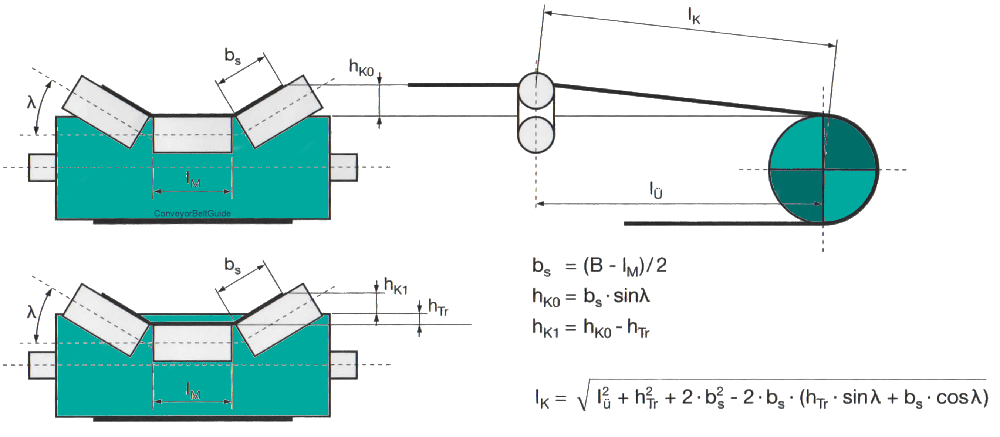Where

qG = conveying mass (kg/m)

H = lift (m)

g = acceleration (m/s²)

SLOPE RESISTANCE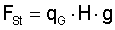TRANSITION CURVES (m)

Where

m'G = length related mass of the conveyor belt (kg/m)

g = acceleration (m/s²)

b = width (mm)

δ = troughing angle

l = idler length (mm)

B = belt width (mm)

Tx = drive traction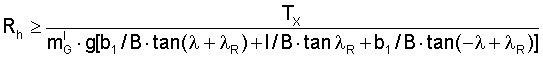Where

Δle = elastic elongation (mm),

Io = initial length of the test piece(mm).

ELASTIC ELONGATION (ISO 9856)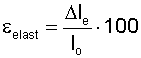Where

Δ lp = permanent elongation (mm),

Io = initial length of the test piece (mm).

Test

PLASTIC (PERMANENT) ELONGATION (ISO 9856)The E-Modulus (Young's modulus) defines the relationship between stress (force per unit area)

and strain (proportional deformation) in a belt,

where

ΔL = amount by which the length changes (mm)

F = force

Ao = original cross-sectional area

Lo = original length (mm)

ELASTIC MODULUS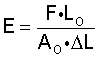Where

F = resistances to motion

v = belt speed

DRIVE POWER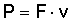Where

FH = primary resistances (idlers, belt indentation, etc.)

FN = secondary resistances (feeding, scrapers etc.)

FS = extraordinary resistances

RESISTANCES TO MOTION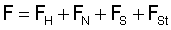Where

FGH is the downhill force

FG is the weight force

Gravity acts straight down (= the weight of the conveyor belt) and the support force acts away from the conveyor. Since the conveyor is sloped, there is a net force acting down the slope.

DOWNHILL FORCE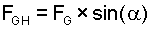The belt friction equation relates the hold-force to the load-force when a belt is is wound around a pulley,

where

e = 2,7183

EYTELWEIN'S EQUATION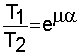The RMS is the square root of the arithmetic mean of the squares of the values.